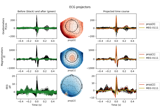# mne.compute_proj_raw#

mne.compute_proj_raw(raw, start=0, stop=None, duration=1, n_grad=2, n_mag=2, n_eeg=0, reject=None, flat=None, n_jobs=None, meg='separate', verbose=None)[source]#

Compute SSP (signal-space projection) vectors on continuous data.

This function aims to find those SSP vectors that will project out the `n` most prominent signals from the data for each specified sensor type. Consequently, if the provided input data contains high levels of noise, the produced SSP vectors can then be used to eliminate that noise from the data.

Parameters:
rawinstance of `Raw`

A raw object to use the data from.

start`float`

Time (in seconds) to start computing SSP.

stop

Time (in seconds) to stop computing SSP. None will go to the end of the file.

duration

Duration (in seconds) to chunk data into for SSP If duration is `None`, data will not be chunked.

n_grad`int` | `float` between `0` and `1`

Number of vectors for gradiometers. Either an integer or a float between 0 and 1 to select the number of vectors to explain the cumulative variance greater than `n_grad`.

n_mag`int` | `float` between `0` and `1`

Number of vectors for magnetometers. Either an integer or a float between 0 and 1 to select the number of vectors to explain the cumulative variance greater than `n_mag`.

n_eeg`int` | `float` between `0` and `1`

Number of vectors for EEG channels. Either an integer or a float between 0 and 1 to select the number of vectors to explain the cumulative variance greater than `n_eeg`.

reject

Epoch PTP rejection threshold used if `duration != None`. See `Epochs`.

flat

Epoch flatness rejection threshold used if `duration != None`. See `Epochs`.

n_jobs

The number of jobs to run in parallel. If `-1`, it is set to the number of CPU cores. Requires the `joblib` package. `None` (default) is a marker for ‘unset’ that will be interpreted as `n_jobs=1` (sequential execution) unless the call is performed under a `joblib.parallel_config` context manager that sets another value for `n_jobs`. Number of jobs to use to compute covariance.

meg`str`

Can be `'separate'` (default) or `'combined'` to compute projectors for magnetometers and gradiometers separately or jointly. If `'combined'`, `n_mag == n_grad` is required and the number of projectors computed for MEG will be `n_mag`.

New in v0.18.

verbose

Control verbosity of the logging output. If `None`, use the default verbosity level. See the logging documentation and `mne.verbose()` for details. Should only be passed as a keyword argument.

Returns:
projs: `list` of `Projection`

List of projection vectors.

## Examples using `mne.compute_proj_raw`#Repairing artifacts with SSP

Repairing artifacts with SSP